JEE  >  Test: Laws of Motion - 3

# Test: Laws of Motion - 3

Test Description

## 30 Questions MCQ Test Physics Class 11 | Test: Laws of Motion - 3

Test: Laws of Motion - 3 for JEE 2023 is part of Physics Class 11 preparation. The Test: Laws of Motion - 3 questions and answers have been prepared according to the JEE exam syllabus.The Test: Laws of Motion - 3 MCQs are made for JEE 2023 Exam. Find important definitions, questions, notes, meanings, examples, exercises, MCQs and online tests for Test: Laws of Motion - 3 below.
Solutions of Test: Laws of Motion - 3 questions in English are available as part of our Physics Class 11 for JEE & Test: Laws of Motion - 3 solutions in Hindi for Physics Class 11 course. Download more important topics, notes, lectures and mock test series for JEE Exam by signing up for free. Attempt Test: Laws of Motion - 3 | 30 questions in 60 minutes | Mock test for JEE preparation | Free important questions MCQ to study Physics Class 11 for JEE Exam | Download free PDF with solutions
 1 Crore+ students have signed up on EduRev. Have you?
Test: Laws of Motion - 3 - Question 1

### A man getting down a running bus, falls forward because-

Detailed Solution for Test: Laws of Motion - 3 - Question 1

The explanation is the question itself. Inertia is a property of which it resists its change of state of rest or state of motion, so as soon as the person jumps the lower part immediately comes to rest by sharing contact with the ground the upper body due to inertia of motion resists its change in state of motion.

Test: Laws of Motion - 3 - Question 2

### You are on a friction less horizontal plane. How can you get off if no horizontal force is exerted by pushing against the surface ?

Detailed Solution for Test: Laws of Motion - 3 - Question 2

Spitting or sneezing this is because you can not jump forward or backward or rolling or even run on the frictionless plane. So you have to throw anything out of your body by applying force Newton's third law follows here and you will escape out.

Test: Laws of Motion - 3 - Question 3

### The forces acting on an object are shown in the fig. If the body moves horizontally at a constant speed of 5 m/s, then the values of the forces P and S are, respectively-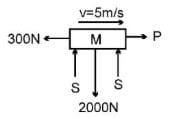Detailed Solution for Test: Laws of Motion - 3 - Question 3

As there is no net acceleration in horizontal or vertical direction, we can say that
P = 300N
And 2S = 2000N
Thus S = 1000N

Test: Laws of Motion - 3 - Question 4

A boy of mass 50 Kg running at 5 m/s jumps on to a 20Kg trolley travelling in the same direction at 1.5 m/s. What is the common velocity?

Detailed Solution for Test: Laws of Motion - 3 - Question 4

Since no external force, law of conservation of momentum can be applied
Initial Momentum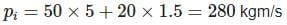Final momentum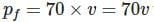where v is the common velocity
Now we know that
Initial momentum = Final momentum
280 = 70v
V = 4m/s

Test: Laws of Motion - 3 - Question 5

Find the velocity of the hanging block if the velocities of the free ends of the rope are as indicated in the figure.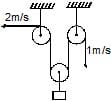Detailed Solution for Test: Laws of Motion - 3 - Question 5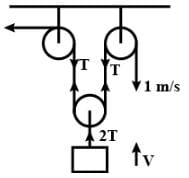Workdone by T = 0
∴ ∑T⋅V = 0
2T + T + 2TV = 0
⇒ − 3T = − 2TV (T & 2T are 180°)
∴ V = 3/2 m/s↑

Test: Laws of Motion - 3 - Question 6

At a given instant, A is moving with velocity of 5 m/s upwards. What is velocity of B at the time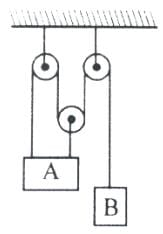Detailed Solution for Test: Laws of Motion - 3 - Question 6

l1 = 2l2 + l= constant
dl1/dt + 2dl2/dt + dl3/dt = 0
−5 + 2(−5) + dl/dt = 0
dl3/dt = 15m/s
⇒ v= 15m/s↓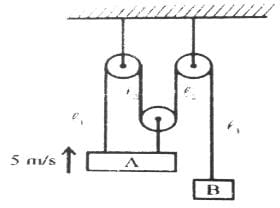Test: Laws of Motion - 3 - Question 7

A body of mass 5 kg is suspended by the strings making angles 60º and 30º with the horizontal -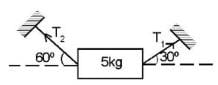(a) T1 = 25 N

(b) T2 = 25 N

(c) T1 = 25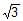N

(d) T2 = 25N

Detailed Solution for Test: Laws of Motion - 3 - Question 7

As the mass is at rest the resultant of forces acting on it are equal to zero so forces in vertical direction are

T1​sin30o + T2​sin60o − mg = 0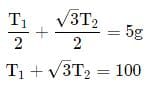similarly in horizontal direction

T1​sin30− T2​sin60= 0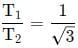solving above equations will give us

T1​+ √3​×√3​T1​ = 100

T​= 25N

T2​ = 25√3​N

Test: Laws of Motion - 3 - Question 8

A cyclist of mass 30 kg exerts a force of 250 N to move his cycle. acceleration is 4 ms−2. force of friction between road and tyres will be

Detailed Solution for Test: Laws of Motion - 3 - Question 8

Net Force = Force exerted by Cyclist - Frictional Force
Also, according to newton's second law
Fnet = m.a
250 N - Frictional Force = 30x4
∴ Frictional Force = 250 - 120 N
= 130 N

Test: Laws of Motion - 3 - Question 9

In the arrangement shown in fig. the ends P and Q of an unstretchable string move downwards with uniform speed U. Pulleys A and B are fixed. Mass M moves upwards with a speed.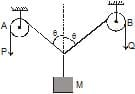Detailed Solution for Test: Laws of Motion - 3 - Question 9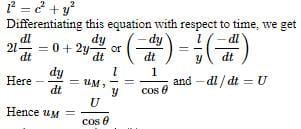Thus option D is correct

Test: Laws of Motion - 3 - Question 10

The velocity of end `A' of rigid rod placed between two smooth vertical walls moves with velocity `u' along vertical direction. Find out the velocity of end `B' of that rod, rod always remains in constant with the vertical walls.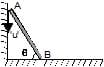Detailed Solution for Test: Laws of Motion - 3 - Question 10

Let say end b has some velocity v in horizontal direction. Thus by constraint motion we get the component of velocities along the rod of both the ends must be equal thus we get,
u.cos (90-q) = v.cos q
Thus we get v = u.tan q

Test: Laws of Motion - 3 - Question 11

A cyclist of mass 30 kg exerts a force of 250 N to move his cycle. The acceleration is 4 ms−2. The force of friction between the road and tyres will be

Detailed Solution for Test: Laws of Motion - 3 - Question 11

As 250N is applied to move the cyclist and cycle, and lets say some frictional force f is acting upon it.
Thus we get 250 - f = 30 x 4 =120
Thus we get f = 130N

Test: Laws of Motion - 3 - Question 12

Find out the reading of the weighing machine in the following cases.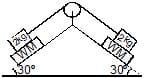Detailed Solution for Test: Laws of Motion - 3 - Question 12

N = mgcosФ
= 2 x 10 x cos30
= 2 x 10 x √3 / 2
= 10√3 N

Test: Laws of Motion - 3 - Question 13

A mass M is suspended by a rope from a rigid support at A as shown in figure. Another rope is tied at the end B, and it is pulled horizontally with a force F. If the rope AB makes an angle q with the vertical in equilibrium, then the tension in the string AB is :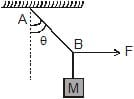Detailed Solution for Test: Laws of Motion - 3 - Question 13

As the block is still at the equilibrium, we get
T.cos q = mg
T.sin q = F
Thus we T = F / sin q

Test: Laws of Motion - 3 - Question 14

Three block are connected as shown, on a horizontal frictionless table and pulled to the right with a force T3 = 60 N. If m1 = 10 kg, m2 = 20 kg and m3 = 30 kg, the tension T2 is-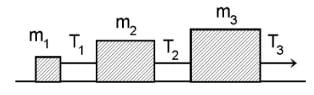Detailed Solution for Test: Laws of Motion - 3 - Question 14

Let a be the acceleration of the system.
T1​ = M1​a  .....(1)
T​− T​= M2​a  ....(2)
F − T2​ = M3​a  ......(3)
Adding (1), (2) and (3)  we get
(M1​ + M2​ + M3​)a = F
or (10+20+30)a = 60
⇒ a = 1m/s2
Now , T2 ​= (M1​+M2​)a
⇒ (10+20)(1) = 30N

Test: Laws of Motion - 3 - Question 15

Three blocks A, B and C are suspended as shown in the figure. Mass of each blocks A and C is m. If system is in equilibrium and mass of B is M, then :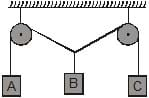Detailed Solution for Test: Laws of Motion - 3 - Question 15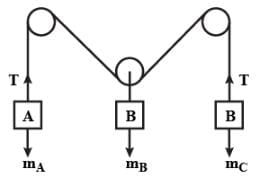Given,
Mass of block A & B = m
Mass of block C = M
Let,
Mass of A = mA​ & Mass of B = mB
Tension in string is = T
At equilibrium, T = mA​g = mB​g = mg
Weight of block C is = Mg
Forces on block C,
2Tcosθ = Mgcosθ
⇒ Mg/2T = Mg/2mg
⇒ ​M/2m
If 0< θ <90° then 1> cosθ >0
1> M/2m >0
2m > M
Hence, 2m > M

Test: Laws of Motion - 3 - Question 16

A weight can be hung in any of the following four ways by string of same type. In which case is the string most likely to break ?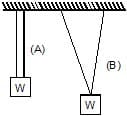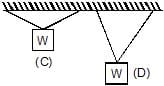Detailed Solution for Test: Laws of Motion - 3 - Question 16
• In all the given cases the cos component of the tension in the string would balance the weight of the block while the sine component will cancel themselves as they are in pair.
• The larger would be the angle the smaller would be the cos component but as its value is fixed i.e. is mg, we get the larger the angle the larger the tension and hence the more chances of breaking of the rope.
Test: Laws of Motion - 3 - Question 17

A force-time graph for a linear motion is shown in figure where the segments are circular. The linear momentum gained between zero and 8 seconds in -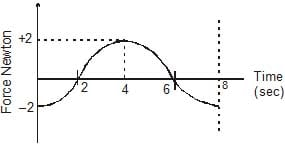Detailed Solution for Test: Laws of Motion - 3 - Question 17

Momentum is rate of change of force according to the given graph initial and final forces are same ie, no change in force therefore b/w 0 and 8 seconds linear momentum is 0.

Test: Laws of Motion - 3 - Question 18

A particle moves in the xy plane under the action of a force F such that the value of its linear momentum (P) at any time t is, Px = 2 cost, Py = 2 sint. The angle q between P and F at that time t will be -

Detailed Solution for Test: Laws of Motion - 3 - Question 18

Fx = dpx / dt = - 2sint
Fy = dpy / dt = 2cost
So angle between F and will be 90º  because we see that their dot product is zero.

Test: Laws of Motion - 3 - Question 19

A stunt man jumps his car over a crater as shown (neglect air resistance)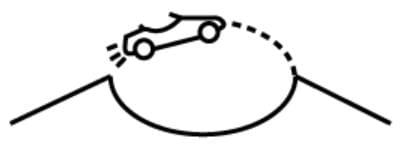Detailed Solution for Test: Laws of Motion - 3 - Question 19
• As the car is in free fall condition it's acceleration will be g downwards.
• Applying pseudo force mg in upward direction , it's weight acts downwards, hence, net force on stunt man is zero and it experience weightless ness.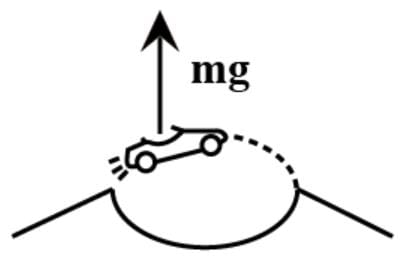Test: Laws of Motion - 3 - Question 20

A particle of mass 50 gram moves on a straight line. The variation of speed with time is shown in figure. find the force acting on the particle at t = 2, 4 and 6 seconds.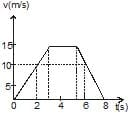Detailed Solution for Test: Laws of Motion - 3 - Question 20
• To know the force acting on the particle, we need to know the acceleration of the particle. In the velocity-time graph the slope of the curve gives the instantaneous acceleration. Let us find it for the given instant.
• At t=2 seconds
The graph is a straight line with positive slope. It means the particle has a constant acceleration with magnitude =15/3 =5 m/s.
So the force acting on it = mass x acceleration = 0.05 kg x 5 m/s
= 0.25 N, it acts along the motion because it is positive.
• At t=4 seconds
The graph is horizontal to time axis meaning thereby the velocity is constant and no acceleration. It can be understood in this way too that the slope which represents acceleration is zero. Since there is no acceleration at t=4 s. so there is no force acting on the particle at this instant, Force= zero.
• At t=6 seconds
The graph shows that velocity is uniformly decreasing with the time and the acceleration which is represented by the slope is negative. From the graph value of acceleration = -15/3 =-5 m/s
Force = mass x acceleration = 0.05 x -5 N =-0.25 N
• So the force acting on the particle is 0.25 N and negative sign shows that its direction is opposite to the motion.
Test: Laws of Motion - 3 - Question 21

Two blocks are in contact on a frictionless table. One has mass m and the other 2m. A force F is applied on 2m as shown in the figure. Now the same force F is applied from the right on m. In the two cases respectively, the ratio force of contact between the two block will be :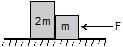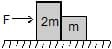Detailed Solution for Test: Laws of Motion - 3 - Question 21
• If F is applied force then acceleration of the system is F/2m + m = F/3m
• Now when we apply the force from the left, the force applied on the block m is F/3m = F/3. This will be the force in the contact.
• When we apply the force from the right from on the block will be 2Fm/3 = 2F/3, this will be the force on the contact then.

So the ratio is F/3 : 2F/3 = 1 : 3

Test: Laws of Motion - 3 - Question 22

A body of mass 8 kg is hanging another body of mass 12 kg. The combination is being pulled by a string with an acceleration of 2.2 m s-2. The tension T1 and T2 will be respectively : (use g = 9.8 m/s2)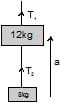Detailed Solution for Test: Laws of Motion - 3 - Question 22

The tension T1 and T2 will be 240 N, 96 N.
Mass of the body = m1 = 12kg (Given)
Mass of the body = m2 = 8kg (Given)
Acceleration of the string - 2.2m/s² (Given)
Considering positive direction upwards -
Thus,
T1− (m1+m2)g = (m1+m2)a
T1 = (m1+m2)g + (m1+m2)a
T1= (12+8)(2.2)+(12+8)(9.8)
T1 = 44 + 196
= 240 N
T2− (m2)g=(m2)a
T2=(m2)g+(m2)a
T2= (8)(2.2)+(8)(9.8)
T2 = 17.6 + 78.4
= 96 N

Test: Laws of Motion - 3 - Question 23

A rope of mass 5 kg is moving vertically in vertical position with an upwards force of 100 N acting at the upper end and a downwards force of 70 N acting at the lower end. The tension at midpoint of the rope is

Detailed Solution for Test: Laws of Motion - 3 - Question 23

When you are given one upward and one downward force on a rope of some mass, and you have to find the tension at the mid- point, then you can simply average the 2 forces. So, the correct answer is (100+70)/3 = 85N

Test: Laws of Motion - 3 - Question 24

A particle of small mass m is joined to a very heavy body by a light string passing over a light pulley. Both bodies are free to move. The total downward force in the pulley is

Detailed Solution for Test: Laws of Motion - 3 - Question 24

The heavy body will fall due to accelaration due to gravity.The accelaration of the lighter body wil be 'g' in upward direction.Taking the equlibrium of the small body

T - mg = ma

but a = g

Therefore

T - mg = mg

T = 2mg

reation at the pulley

2T = 4mg

Test: Laws of Motion - 3 - Question 25

The pulley arrangements shown in figure are identical the mass of the rope being negligible. In case I, the mass m is lifted by attaching a mass 2 m to the other end of the rope. In case II, the mass m is lifted by pulling the other end of the rope with cosntant downward force F = 2 mg, where g is acceleration due to gravity. The acceleration of mass in case I is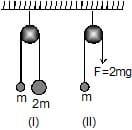Detailed Solution for Test: Laws of Motion - 3 - Question 25

When we make the free body diagram of two mass system in case 1, we get the equation
2mg - mg = (2m + m) a
Thus we get a = g/3
But in the case 2 the equations is as follows
2mg - mg = ma
Thus we get a - g here.

Test: Laws of Motion - 3 - Question 26

Two masses M1 and M2 are attached to the ends of a light string which passes over a massless pulley attached to the top of a double inclined smooth plane of angles of inclination a and b. The tension in the string is: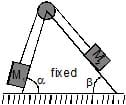Detailed Solution for Test: Laws of Motion - 3 - Question 26

If we straighten the string horizontally hypothetically and make the free body diagram for the whole two block system we get that
M1g.sinɑ - M2g.sinβ = (M1 + M2)a
For some net acceleration of the system a
Such that a = M1g.sinɑ - M2g.sinβ / (M1 + M2)
Hence if we make a free body diagram of mass 1 only we get
M1g.sinɑ - T = M1a
Thus we get T = M1 ( g.sinɑ - a )
= M1( g.sinɑ - [M1g.sinɑ - M2g.sinβ / (M1 + M2) ] )
= M1( M2 (sinɑ + sinβ )f / (M1 + M2) )

Test: Laws of Motion - 3 - Question 27

Two masses are hanging vertically over frictionless pulley. The acceleration of the two masses is-

Detailed Solution for Test: Laws of Motion - 3 - Question 27

Free Body Diagram: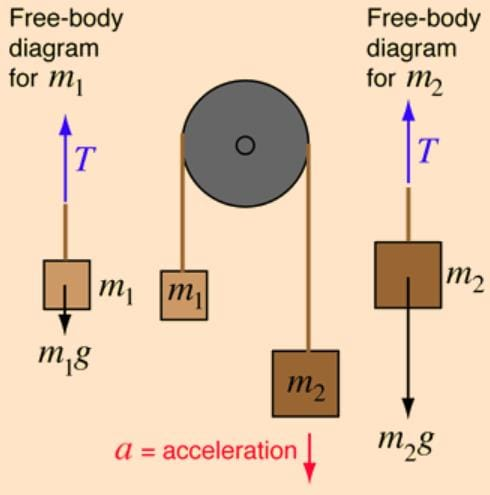Fnet = m2g - m1g
F = (m+ m2) a
m2g - m1g = (m+ m2) a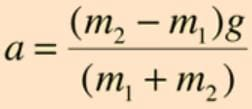Test: Laws of Motion - 3 - Question 28

Three equal weights A, B, C of mass 2 kg each are hanging on a string passing over a fixed frictionless pulley as shown in the fig. The tension in the string connecting weights B and C is-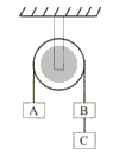Detailed Solution for Test: Laws of Motion - 3 - Question 28

For some time let us consider B and C to be one single block of mass 4kg. Now if we make the free body diagram for blocks A, B, C we get the net acceleration of the system by equation
4.g - 2.g = 6.a
Thus we get a = g/3
Now if we only make a F.B.D. of block C, we get
2.g - T = 2.a
Thus T = 2 (g - g/3)
= 4g/3
= 13.3 N

Test: Laws of Motion - 3 - Question 29

In the arrangement shown in figure, pulleys are massless and frictionless and threads are inextensible. The Block of mass m1 will remain at rest, if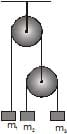Detailed Solution for Test: Laws of Motion - 3 - Question 29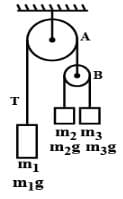a = [(m3​−m2 )/(m2​+m3​) ​​]g  (m> m2)
T = [2m2​m3​g] / [m2​+m3] ​
​T′ = 2T = [4m2​m3​g​] / [m2​+m3​]
m1​g = 4m2​m3​g​m / m2​+m3
4/m1​ = [1/m2​​] + [1/m3​]​​

Test: Laws of Motion - 3 - Question 30

A block is placed on a rough floor and a horizontal force F is applied on it. The force of friction f by the floor on the block is measured for different values of F and a graph is plotted between them-

Detailed Solution for Test: Laws of Motion - 3 - Question 30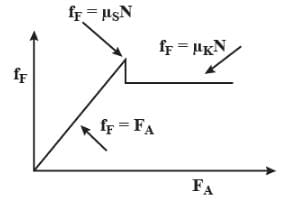For a small value of the applied force the force of friction f increases linearly upto the limiting friction. When it crosses the maximum point of static friction then the friction force due to kinetic friction does not change any more.

## Physics Class 11

127 videos|464 docs|210 tests
 Use Code STAYHOME200 and get INR 200 additional OFF Use Coupon Code
Information about Test: Laws of Motion - 3 Page
In this test you can find the Exam questions for Test: Laws of Motion - 3 solved & explained in the simplest way possible. Besides giving Questions and answers for Test: Laws of Motion - 3, EduRev gives you an ample number of Online tests for practice

## Physics Class 11

127 videos|464 docs|210 tests